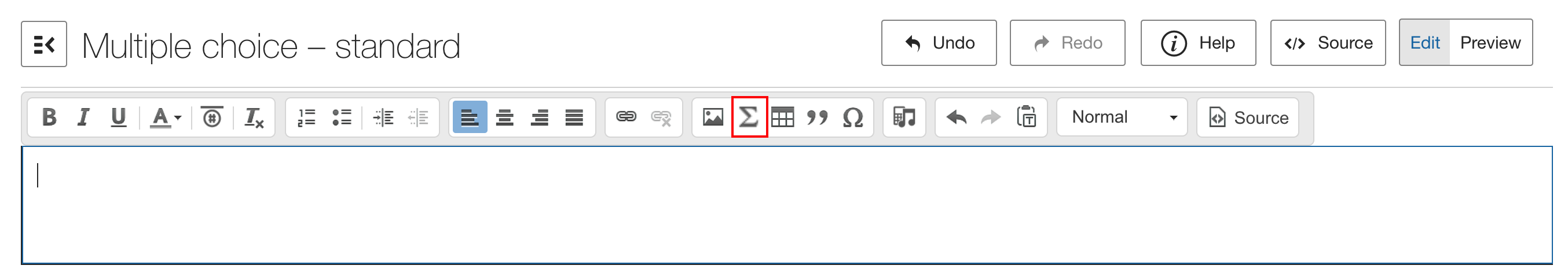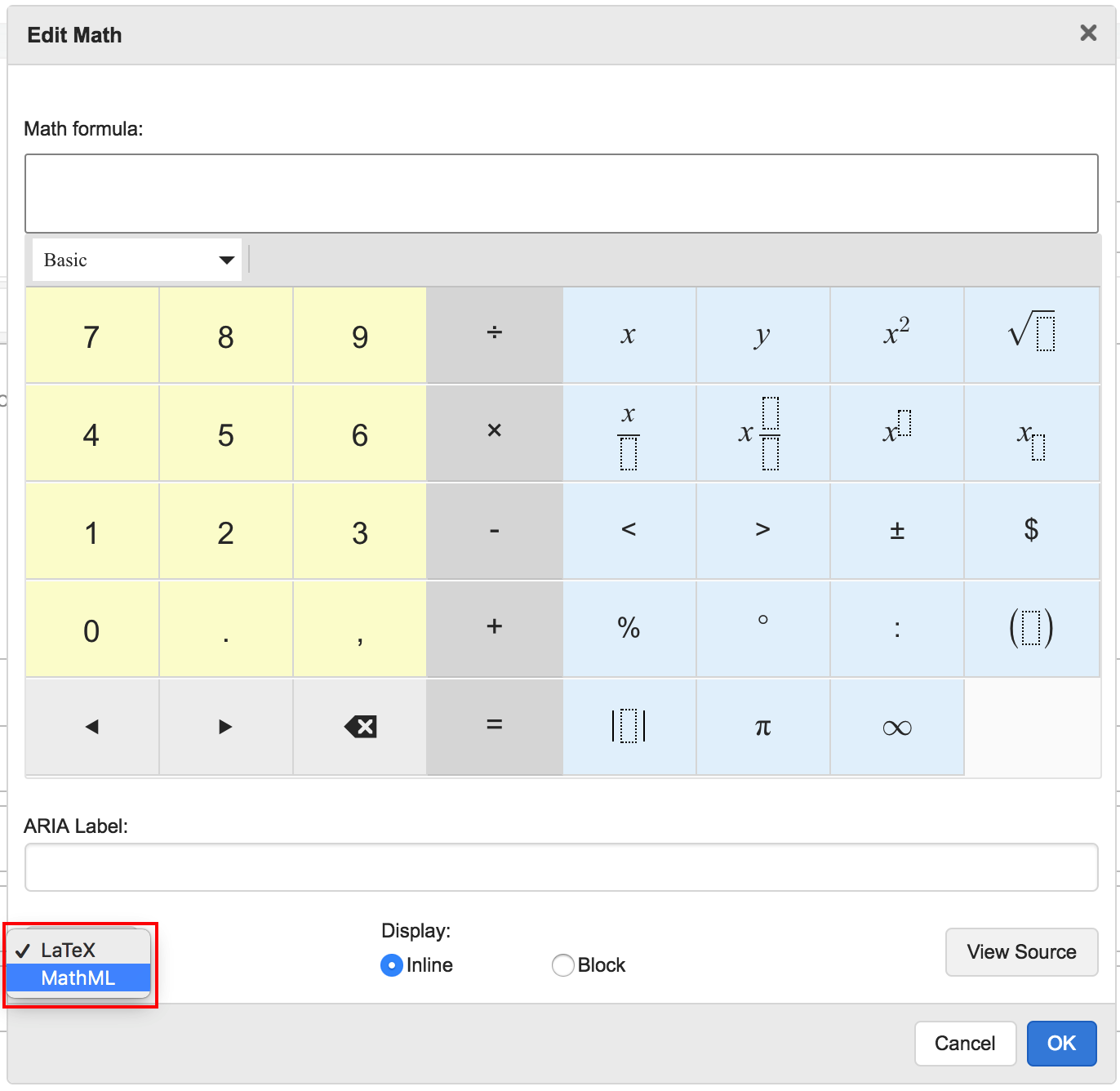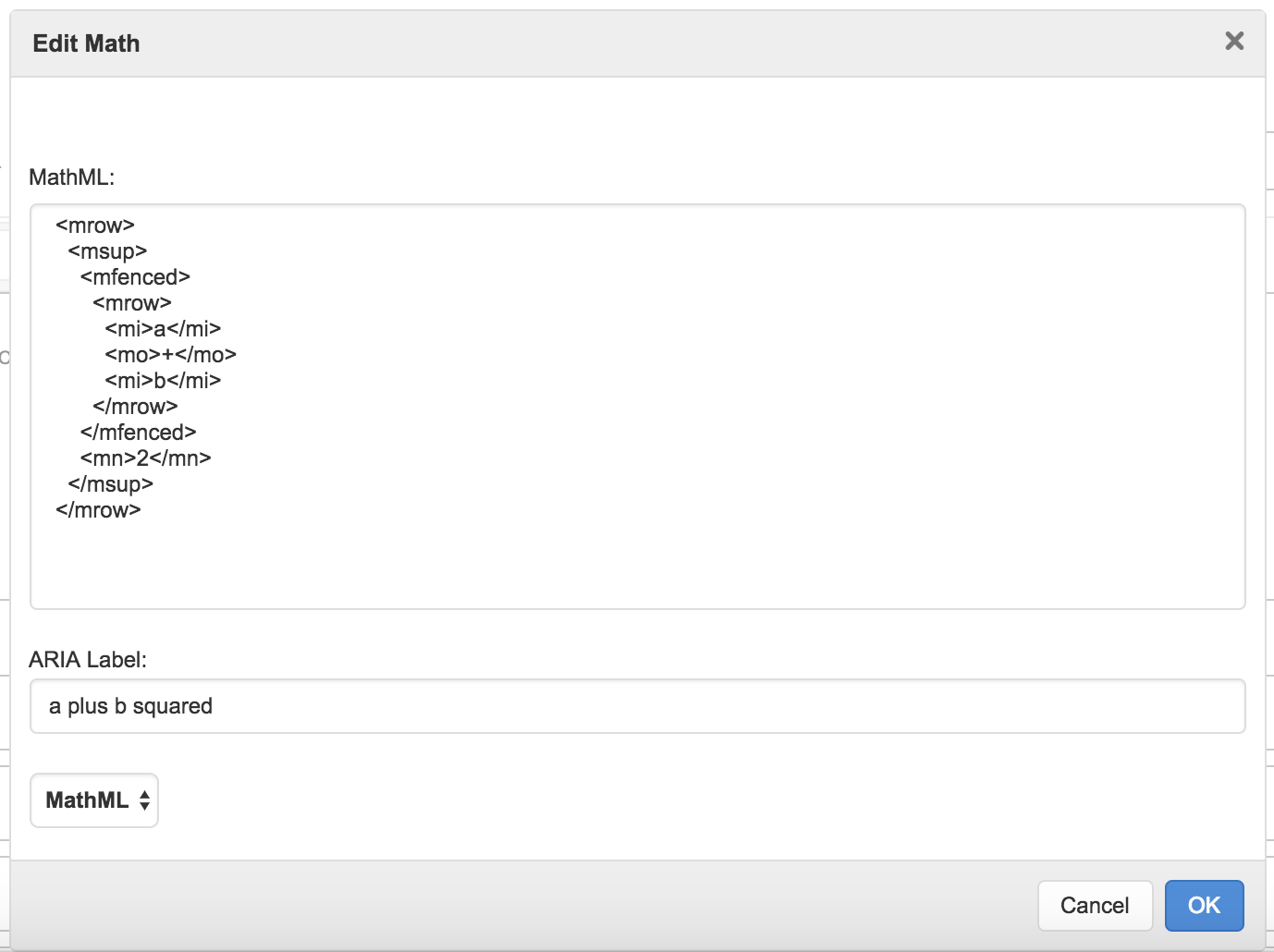Inserting MathML into a Question using the Rich Text Toolbar's Math Editor

Our Math Editor allows you the author to input Math symbols in any Question that contains rich text fields. This means you can mix questions like Multiple Choice and any of our drag and drop options with math symbols, expanding the kinds of math questions you can ask.

The Math Editor can be found in any field that contains the rich text toolbar, and is denoted by the uppercase sigma symbol:Figure 1: The Rich Text Toolbar in the Compose Question field of an MCQ.

By default, the math markup language used by the Math Editor is set to LaTeX. To change from LaTeX to MathML, select MathML from the drop down menu in the bottom right of the Editor.Figure 2: Select MathML from the drop down menu.

Now you will be able to enter math markup in the MathML field. Underneath, you can manually enter an ARIA Label - this can be edited at any time.Figure 3: MathML markup and ARIA label fields.